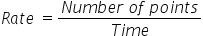Mathematics
Easy

Question

# Ryan scored 104 points in 26 minutes at a quiz competition. At the same rate, _______ points are scored in 40 minutes.

## 140160135125Hint:

## The correct answer is: 160

### Ryan scored 104 points in 26 minutes.We have to find the rate at which Ryan scores the points. We will find it by taking the ratio of the number of points and the time required to score it. As the rate is constant, we will use this value to find the number of points scored in 40 minutes.So the equation becomesSo the rate = 4 points per minute.Let’s find the number of points in 40 minutes.We will rearrange this equation for the number of points,as we have to find the number of points.For this we will multiply both sides by time.Time × Rate = Number of pointsNumber of points = Time × RateSubstituting the values we have foundNumber of points = 40 × 4Number of points 160Therefore, number of points scored by Ryan in 40 minutes is 160.

We should be careful about the units. We should make sure that same quantities have same value.

### Related Questions to study#### With Turito Foundation.#### Get an Expert Advice From Turito.